# What Matters for Driving Distance?

Dave Tutelman  -  June 5, 2012

I received an email from Reinout Schotman saying:
In golf, as you know, many dogmas (mantras) exist, which are not properly supported by data.

I downloaded some performance data of PGAtour.com and did some assessments.

Surprisingly I found no (statistically significant) correlation between Launch Angle and total distance and neither for Spin and total distance. The only thing that seems to matter is to hit it hard (swing speed) and on the sweet spot (smash factor). And obviousely straight and in the right direction...

The dogma "High Launch, Low Spin" does not seem to hold, at least for PGA Tour Pro's. Is this known?

I have data and graphs in Excel to support, if you're interested.
In support of his conclusion, Reinout presents the following scatter plots, along with a best-straight-line fit to the data. (Click on the thumbnail for a full-size view.)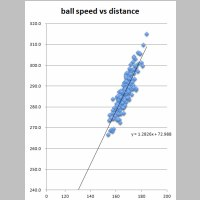Ball speed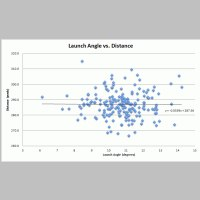Launch Angle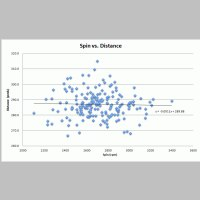Spin

I am not much surprised by Reinout's conclusion. He has correctly identified the three launch parameters as the primary determinants of driving distance:
• Ball speed (a combination of clubhead speed and smash factor)
• Launch angle
• Spin
Of the three, it is clear (to me at least) that ball speed is by far the most important. Given a ball speed that a player can produce, launch angle and spin tend to be just tweaks to the distance. For more detail, see my article on driver optimization. To get further confirmation from the statistics, let's look at the trend line. Reinout drew his conclusion about lack of correlation from the slope of the trend line; the larger the slope, the greater the correlation. But even more telling is the R-squared value for the line; it is a value that measures the statistical significance of any correlation you notice. (Zero means no correlation, and one means perfect correlation.) Here are the slopes and the R-squared values for our three launch parameters:

 Ball Speed Launch Angle Spin Slope 1.3 yards per mph -.06 yards per degree -.001 yards per rpm R-squared .76 .00008 .0008

We see ball speed has an advantage not only in slope but in the statistical significance of that correlation. And not just a small advantage either; the advantage is quite a few orders of magnitude in both slope and R-squared.

So the Tour stats say that ball speed affects distance way more than does launch angle or spin. But can we explain it -- can we describe it more in mechanical terms than statistical? Good challenge!

## A Five Percent Solution

Reinout's conclusion was for total distance -- though the PGAtour.com data also includes carry distance. I have a computer simulation tool that can answer the question for carry distance: the TrajectoWare Drive computer program. Let's see how each of these parameters affects the carry distance. We will also note the angle of descent, which is the biggest single factor in how far the ball runs after landing.

We will assume a fairly typical PGA Tour golfer. He has a clubhead speed of 115mph and uses a driver with a 10º loft. If we plug his impact into the computer program, we get:

 Launch Parameters Results Ball Speed Launch Angle Spin Carry Distance Descent Angle 168.5 mph 8.9º 3195 rpm 277.2 yd 38.3º

These will be our reference values, and we will see how they vary as we vary the launch parameters. We will change the launch parameters up and down by 5% of their value.

 5% down 5% up Total Variation Parameter value Carry Distance Descent Angle Parameter value Carry Distance Descent Angle Carry Distance Descent Angle Ball Speed 160.1 mph 260.0 36.0 176.9 mph 293.4 40.5 33.4 4.5 Launch Angle 8.5º 276.2 37.6 9.4º 278.3 39.3 2.1 1.7 Spin 3035 rpm 277.1 37.0 3355 rpm 276.7 39.7 -0.5 2.7

What do we see here? Ball speed is fifteen times as important as anything else in producing carry distance. That is the simple confirmation of Reinout's observation. If I were looking at data from tour players instead of a carefully controlled computer "experiment", the player-to-player variation would swamp out any effect of launch angle or spin. Only ball speed would show up as a statistically significant factor.

But that is just carry distance. Could launch angle or spin produce enough variation in runout after landing to affect the statistics? It doesn't look like it. True, ball speed has the biggest effect on the angle of descent, and a high ball speed will result in a steep descent, limiting rollout. But it is not going to come close to any significant effect on the comparison; the carry-distance advantage of ball speed is overwhelming.

## A Standard Deviation

Reinout looked at the table above and said that I was giving too much weight to ball speed. A 5% variation in ball speed is very difficult to accomplish, whereas one can effect much larger changes than 5% in launch angle and spin. He has a point! So I repeated the calculation, using a carefully considered variation for each of the three launch parameters.

What I did was compute the average and standard deviation for each of the parameters. This was not hard, because Reinout had already transferred the data from PGAtour.com to an Excel spreadsheet that he shared with me. I just had to add a row for standard deviation; he already had computed the averages. The spreadsheet contained data from 186 PGA Tour players, and included the three launch parameters, carry distance, and total distance (among others, but angle of descent was not included). Below is a table reflecting the bulk statistics.

 Launch Parameters Results Ball Speed Launch Angle Spin Carry Distance Descent Angle Average 167 mph 10.7º 2700 rpm 273 yd No Data Standard Deviation 5.6 mph 1.3º 222 rpm 11.5 yd Percentage 3.4% 12.1% 8.2% 4.2%

I have also included a percentage, which is the standard deviation as a fraction of the average. This number confirms Reinout's contention; a blanket ±5% will give misleading values. Ball speed (the dominating parameter in our first try) has a standard deviation less than 5%, while launch angle and spin are both more than 5%.

So... Let's repeat the table of sensitivities we calculated above, but this time with the "Average" values as our reference values, and vary them up and down by an amount equal to the standard deviation. That should give us a perfectly representative set of carry distance variations, for the 186 players in the data.

(Note that the computer model gives a carry distance of 278.2 yards for the launch conditions in the "Average" row. That is not the same as the average carry distance in the data: 273 yards. We are not yet in a position to discuss this discrepancy; we'll just accept it and do the math. For now, anyway; we'll get back to it later.)

 1 Std. Dev. down 1 Std. Dev. up Total Variation Parameter value Carry Distance Descent Angle Parameter value Carry Distance Descent Angle Carry Distance Descent Angle Ball Speed 161.4 mph 266.5 36.0 172.6 mph 289.6 38.9 23.1 2.9 Launch Angle 9.4º 274.2 34.9 12º 281.5 39.8 7.3 4.9 Spin 2478 rpm 277.0 35.8 2922 rpm 278.6 39.1 1.6 3.3

The ball speed still dominates, but not by as much as before. Instead of being 15 times as important, it is only three times as important as launch angle. But that is still a large margin. So the conclusion still stands.

While we are looking at the data, Reinout's original conclusion was that launch angle is not significantly correlated to distance. This table says that, while ball speed is three times as important, there should be a non-negligible effect from launch angle. Why did Reinout conclude otherwise? An answer may lie in the descent angle. Note that:
• Our table deals with carry distance.
• Reinout's conclusion is for total distance.
In our new table, we have amped up the relative effect of launch angle. The first table had ball speed and launch angle at the same percentage difference; this new table has launch angle variation more than three times that of ball speed. As a result, launch angle variation gives almost twice as much difference in angle of descent as does ball speed. And angle of descent hurts rollout after landing. Let's check this hypothesis against the data -- which has columns and graphs for both carry distance and total distance. Here are two graphs from Reinout's spreadsheet, showing the correlation between launch angle and distance.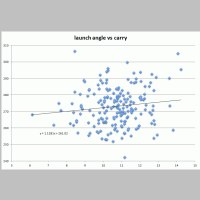Carry Distance vs Launch AngleTotal distance vs Launch Angle

This pair of scatter plots provides a very interesting result! Specifically, look at the best-fit straight line and its slope. There is a significant slope to the carry distance line: about an extra yard of carry per degree of added launch angle. But this slope disappears completely when we fit total distance to launch angle. This says that there is a substantial negative correlation between launch angle and rollout after landing. When we raise the launch angle we may improve carry distance, but we do nothing for total distance. And that observation is readily explained by our conjecture about angle of descent.

## A Fitting Observation

Let's recognize a very important fact: Tour players all have drivers that were fitted to their swings by expert clubfitters. Not one of them plays with a random, off-the-shelf driver, but rather a driver optimized to that golfer.

This fact is fairly important in evaluating the effect of launch conditions on distance, based on Tour players' performance. Two important points:
• It is pretty obvious that, all other things being equal, additional ball speed turns into additional distance. A properly fitted driver means there is nothing getting in the way of clubhead speed turning into ball speed turning into distance. So let's not even consider the effect of ball speed; that's a given. And it is easily confirmed by playing with the computer model, or by noting that the statistical correlation is very strong (R-squared is well on its way to one; it is .76).
• For the rest, we need to understand how a proper fitting relates to the computer model. Let's look closer at this.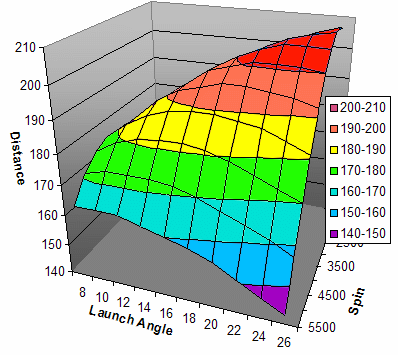Here is a picture of the "launch space" for a clubhead speed of 86mph. It is a three-dimensional graph of distance for values of launch angle and spin. The resulting surface is like a sheet of paper that has been bent down at two diagonally opposite corners. (Those corners are high-launch/high-spin and low-launch/low-spin. The turned-down corners are obviously short carry distances, where nobody would ever want to design a driver.) There is a round "ridge" of maximum distance, lying diagonally across the other two corners, and that ridge is sloped slightly upwards toward the high-launch/low-spin corner. A properly fit driver should be designed to lie along this ridge, for the golfer being fit. I mentioned that this example represents a clubhead speed of 86mph. That is not a Tour clubhead speed. I have played with these graphs for golfers from senior women to long-drive competitors. The shape is always the same; only the numbers on the axes change. That is, a Tour golfer's graph would have lower launch angles, lower spin, and higher carry distance. But it would still have those two corners tucked down, and a slanted ridge across the other two corners. Because the shape is the same, we can learn from this graph what we need to know about the fitting process. (I had this graph on hand from existing research, and didn't want to bother going through the tedious work involved in calculating and creating another publication-quality launch space graph.) Fitting a golfer for a driver involves finding a set of components that work for that golfer's swing. The fitting parameters include characteristics of both the club and the golfer, and include: Loft. Club length. Weight and balance. Shaft flex and also flex profile (how the sitffness changes over the length of the shaft). The golfer's clubhead speed. The golfer's angle of attack, and wrist bowing or cupping at impact. The golfer's ability to repeat the same swing; for Tour players, this tends to be very good. The savvy clubfitter will start with a rough cut at loft, and optimize everything else. Then he will turn back to loft, and find the ideal loft for the golfer. This process can be looked at in launch space. (Though I will admit that I know of no professional clubfitter who actually looks at driver fitting as a launch space graph.)Here is another look at the 86mph launch space, with some clubfitting information added. First of all, the "ridge" of maximum distance is a red dotted line. Then a sequence of real drivers with various lofts are plotted in black. We can do this because, knowing the loft and the clubhead speed, we can compute the launch angle and spin. For instance, a driver with a fourteen-degree loft swung at 86mph will propel the ball at a launch angle of 12º with a spin of 3300rpm. So we plot a black dot on the surface at [12º, 3300rpm] and label it "14". (And we see that the carry distance is 188yd, the height of the launch surface of the graph at that point.) I computed points from 8º to 24º, and show them on the graph as black dots connected by a black line. This is the line of feasible performance for the driver of someone who swings the driver at 86mph at a 0º angle of attack. We can now see fitting that golfer for driver loft as a mathematical process: find the highest point on the black curve, and note the loft that point corresponds to. Think about this representation of fitting a golfer for driver loft. Get comfortable with visualizing it on a 3D graph like the one above. What follows depends on it. Any optimization of a continuous function (like the black curve in the graph) involves finding a place where the curve is horizontal; it doesn't go up or down as you change where you are by small amounts. If you look at the height of the curve (the carry distance) for lofts of 14º, 15º, and 16º, you see distances of 188, 189, and 188 yards respectively. That is almost no change at all. The basic mathematical approaches to optimization depend on this. They look for a "flat spot" in the function. In this case, we are looking for a flat spot in the funtion of carry distance vs loft -- the black line. The middle of the flat spot is 15º, so that is what our optimum driver should be. Now take a look at the surface near the maximum carry distance. The ridge is only sloped a little bit, near the flat spot in the loft curve. That means that distance is flat not just with loft, but also with launch angle and spin. Let's put this back into Reinout's observation. Since the Tour players have well-fitted drivers, their performance in launch space does not change much with launch angle and spin. This is not the case everywhere in launch space. It is definitely the case at the flat part of the curve -- where a well-fitted driver lives. But let's look at a really poorly fitted driver and see if that is still the case. Consider our 86mph swinger trying to use a 24º driver; in this portion of the surface, small changes in spin give large changes in distance. That is very different from what Reinout observed. For the maximum-carry loft of 15º, a change of 500rpm of spin gives a difference of only a yard or two. For a 24º driver (the "front edge" of the surface), a change of 500rpm gives a difference of twelve yards, certainly a significant difference. The same turns out to be true for too-low a loft; for an 8º driver, a change of 500rpm again gives a difference of twelve yards. So Reinout's observation depends on a reasonably well-fitted driver, certainly a reasonable assumption for a Tour player. And, if your own driver is so ill-fitted that spin and launch angle make big differences in your distance, you should be getting a new, properly fit driver post haste.

## Conclusion

Reinout Schotman has observed that, statistically from current PGA Tour data, ball speed is a significant factor in driving distance, but launch angle and spin are somwhere between insignificant and zero.

In this article, we have seen that:
• This is indeed an accurate statement.
• Computer modeling agrees that is the way it should be.
• This is partly the result of Tour pros using the right driver that fits them. If the driver were a really bad fit, then spin would be a significant factor (and perhaps launch angle as well, though we didn't explore that here).

## Math addendum: Is this really valid?

Here's a mathematical fine point that you may or may not be interested in. If you're not into math, you don't need to understand -- nor even read -- this note. Skip it if it holds no interest for you.

While I was working on answering Reinout's question, I started to wonder whether it is valid to compare a deterministic computer/physics model with the type of statistical model Reinout gleans from the PGAtour.com statistics. It seems to give reasonable answers, but it is mathematically suspect. Here's the problem; the statistical model and the computer model are not the same, so it may not be extremely important that they give the same answers.
• The computer model takes a set of launch conditions, and computes a carry distance that physics says those launch conditions will produce.
• The statistical model takes a set of data points, each of which is an average of a season's worth of swings for one single professional golfer. We then look at the distribution of those points. That is, the carry distance for Bubba Watson's row on the spreadsheet is the average of all his drives, the ball speed is the average of all his drives, etc. All those drives are repesented as a single point in the scatter plots -- each point is the statistical summary for one player.
It may seem frivolous to question testing a deterministic mathematical model with a statistical model. In science, theories are tested that way all the time. When there is any randomness or outside influences in the experimental results, scientists turn to the sort of graphs Reinout presented. But this is substantially different. If we were using statistics to test the mathematical model behind TrajectoWare Drive, the statistical base would have one point per measured drive -- not one point representing a season's worth of measured drives.

What does this do to the statistics we observe? At the very least, it is probably making R-squared much smaller than it should be. If each data point were a single drive, I would expect the trend line slopes to be pretty similar to what we see. But I would expect the points to line up much better along that line, not scatter all over the page. And that would result in a much better correlation of the random effects in the experiment, an R-squared closer to 1.0.

Why do we see so much spread in the data? Because even a single player's driving statistics are not uniform. For instance, data will be taken on holes where the player used a driver (certainly the intent of Reinout's study), but also on holes where he used a 3-wood, or perhaps even an iron. Uphill and downhill. Into the wind, with the wind, crosswind, and combinations thereof. What do we get when we average all those drives into a single point? I honestly have no idea. And that is exactly the problem!

Think about this: When Reinout does the statistical curve fitting, he is tacitly assuming that the statistical fit will reflect repeated use of the single-instance computer model. But that assumption is mathematically valid only if the computer model is linear. If the model is nonlinear, then the distributions are warped by the nonlinearity, and the average of the computed carries will not necessarily be the measured average of carries. But we know (looking at the launch space surface) that the function of carry distance for ball speed, launch angle, and spin is not linear. Perhaps the restriction of launch space implied by properly fitted drivers keeps us in a region where the function is close enough to linear that the linearity assumption does not do any damage.

So the fact that the computer model continue to give the same information as the statistics might be coincidence. More likely, it is a rough approximation to what we would get if we gathered the statistics properly -- one drive per data point. Either way, we got lucky.

### Notes:

1. That is why calculus is used for optimization; the first derivative of a function is its slope. To find a maximum or minimum of the function, we differentiate the function. Then we set the derivative to zero and solve for x (and/or y, z, etc). The values of [x, y, z] where the derivatives are zero is the maximum or minimum of the function.
2. Actually, that is an oversimplification -- but it is a good starting point. For instance, I usually back off about a degree -- from 15º to 14º in this case -- to give up a little carry in favor of runount, because I know lower loft gives lower angle of descent.

Last modified -- June 16, 2012